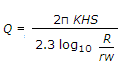# Civil Engineering - Water Supply Engineering

### Exercise :: Water Supply Engineering - Section 4

6.

If the average daily demand of a city of 50, 000 population, is 20 m.l.d., the maximum daily demand is

 A. 24 mld B. 30 mld C. 36 mld D. 54 mld.

Explanation:

No answer description available for this question. Let us discuss.

7.

Recuperation test is carried out to determine

 A. turbidity of water B. pH value of water C. yield of well D. discharge from a well.

Explanation:

No answer description available for this question. Let us discuss.

8.

The dischargefor the confined tube well is obtained from

 A. Thiem's formula B. Darcy's formula C. Tolman's formula D. Dupuits formula.

Explanation:

No answer description available for this question. Let us discuss.

9.

Pick up the correct statement from the following :

 A. Air lift pumps are generally used for pumping water from deep wells B. Jet pumps are generally used for pumping water from small wells C. The hydraulic ram works on the principle of water hammer D. Rotary pumps are provided cams which rotate in opposite direction E. all the above.

Explanation:

No answer description available for this question. Let us discuss.

10.

Turbidity of water is expressed

 A. in ppm B. in numbers in an arbitrary scale C. by pH value D. by colour code.